• [单链表]一元多项式定义求和
• 输入多项式项数后，对多项式进行描述，将求两个多项式和
• 仅为demo基础版，可拓展。
• 单链表实现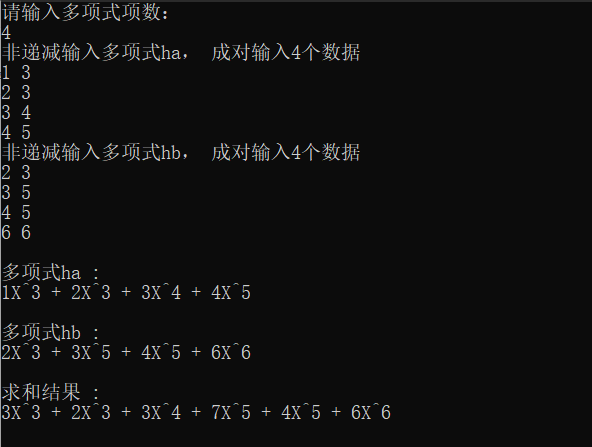#include<stdio.h>
#include<stdlib.h>
#include<malloc.h>
typedef int ElemType;
/*单项链表的声明*/
typedef struct PolynNode{
int coef; // 系数
int expn; // 指数
struct PolynNode *next;
}PolynNode,*PolynList;
/*正位序(插在表尾)输入n个元素的值，建立带表头结构的单链线性表*/
/*指数系数一对一对输入*/
void CreatePolyn(PolynList &L,int n){
int i;
PolynList p,q;
L=(PolynList)malloc(sizeof(PolynNode)); // 生成头结点
L->next=NULL;
q=L;
printf("成对输入%d个数据\n",n);
for(i=1;i<=n;i++){
p=(PolynList)malloc(sizeof(PolynNode));
scanf("%d%d",&p->coef,&p->expn);    //指数和系数成对输入
q->next=p;
q=q->next;
}
p->next=NULL;
}
// 初始条件：单链表L已存在
// 操作结果: 依次对L的每个数据元素调用函数vi()。一旦vi()失败,则操作失败
void PolynTraverse(PolynList L,void(*vi)(ElemType, ElemType)){
PolynList p=L->next;
while(p){
vi(p->coef, p->expn);
if(p->next){
printf(" + ");   //“+”号的输出，最后一项后面没有“+”
}
p=p->next;
}
printf("\n");
}
/*ListTraverse()调用的函数(类型要一致)*/
void visit(ElemType c, ElemType e){
if(c != 0){
printf("%dX^%d",c,e);   //格式化输出多项式每一项
}
}
/*    多项式相加，原理：归并        */
/* 参数：两个已经存在的多项式       */
/* 返回值：归并后新的多项式的头结点 */
PolynList MergeList(PolynList La, PolynList Lb){
PolynList pa, pb, pc, Lc;
pa = La->next;
pb = Lb->next;
Lc = pc = La;   // 用La的头结点作为Lc的头结点
while(pa&&pb){
if(pa->expn < pb->expn){
pc->next = pa;             //如果指数不相等，pc指针连上指数小的结点，
pc = pa;
pa = pa->next;             //指向该结点的指针后移
}
else if (pa ->expn > pb->expn ){
pc->next = pb;               //pc指针连上指数小的结点，
pc = pb;
pb = pb->next;               //指向该结点的指针后移
}
else{   //(pa ->expn = pb->expn )

pa->coef = pa->coef + pb->coef;     //指数相等时，系数相加
pc->next = pa;
pc = pa;
pa = pa->next;             //两指针都往后移
pb = pb->next;
}
}
pc->next = pa ? pa:pb;  // 插入剩余段
return Lc;
}

int main(){
PolynList ha,hb,hc;
int a;
printf("请输入多项式项数：\n");
scanf("%d",&a);

printf("非递减输入多项式ha， ");
CreatePolyn(ha,a);   // 正位序输入n个元素的值

printf("非递减输入多项式hb， ");
CreatePolyn(hb,a);   // 正位序输入n个元素的值

printf("\n");

printf("多项式ha :\n");
PolynTraverse(ha, visit);
printf("\n");
printf("多项式hb :\n");
PolynTraverse(hb, visit);
printf("\n");

printf("求和结果 :\n");
hc = MergeList(ha,hb);
PolynTraverse(hc, visit);  }

展开全文数据结构
• 一，有序齐次单项式空间2阶有序...我们观察二阶有序齐次单项式，在H中做内积有： 我们定义函数： 由此我们推算到d阶的有序齐次单项式： 二，有序单项式空间类似的可以推导出，二阶有序单向式空间： 类似的推导到...

一，有序齐次单项式空间

2阶有序齐次单项式：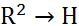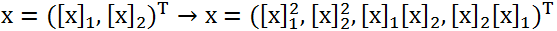由此推广，可以得到d阶的有序齐次单项式：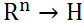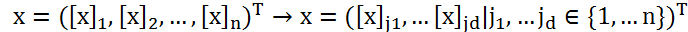由上式可知，如果n和d数据过大时，内积的运算量将会非常大。

我们观察二阶有序齐次单项式，在H中做内积有：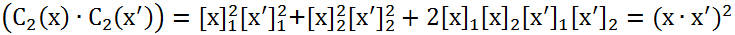我们定义函数：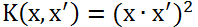由此我们推算到d阶的有序齐次单项式：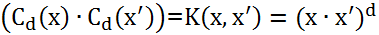二，有序单项式空间

类似的可以推导出，二阶有序单向式空间：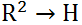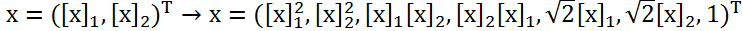类似的推导到多阶：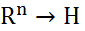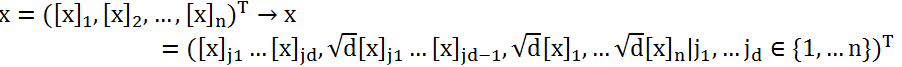类似推定义函数：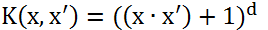推算到d阶的有序单项式：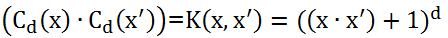三，无序单项式空间

在上述有序的单项式中，我们把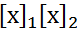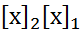看做不同的分量而区分，而实际上我们可以把它看做是无序的，相等的，将映射调整为：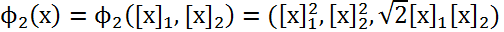仍然可以得出：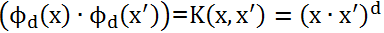四，Hilbert空间与多项式核函数

由前面可知支持向量分类机只与内积有关系，而与映射到的Hilbert空间并无直接联系，所以以后我们只关系内积。我们把此函数统称为多项式核：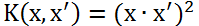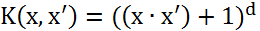例子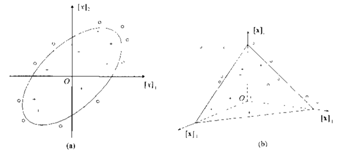假设一个椭圆可以对类进行划分（如图（a））：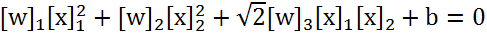引入非线性映射：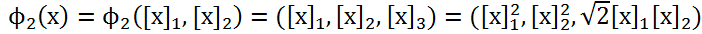获得三维空间，使用支持向量机分划超平面（如图(b)）: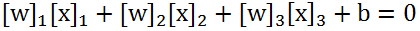展开全文•   设 a0,a1,…,an都是数域 F 中的数， n 是非负整数，那么表达式anxn +an-1xn-1+…+a2x2 +a1x1+ a0x0(an≠0) 叫做数域 F上一个文字 x 的多项式或一元多项式。   在多项式中，a0叫做零次多项式或常数项，a1x 叫做...

一、基本概念

设 a0,a1,…,an都是数域 F 中的数， n 是非负整数，那么表达式anxn +an-1xn-1+…+a2x2 +a1x1+ a0x0(an≠0) 叫做数域 F上一个文字 x 的多项式或一元多项式
在多项式中，a0叫做零次多项式或常数项，a1x 叫做一次项，一般，aix 叫做i次项，ai 叫做 i 次项的系数。一元多项式用符号 f(x)，g(x)，…来表示。
一元稀疏多项式： 在一元多项式中，若系数为0的项数目(也就是不存在的项，指的是比最高次低的项)远远多于非0项的数目(存在的项)，并且非0项分布没有规律时，则称该一元多项式为一元稀疏多项式。

二、问题描述

1. 输出多项式，输出形式为整数序列：n,a1,e1,a2,e2,…,an,en，其中n是多项式的项数，ai,ei分别是第i 项的系数和指数，序列按指数降序排列。
2. 实现多项式a和b相加。
3. 实现多项式a和b相减。
4. 实现多项式a和b相乘。

输入举例：
7-5x2+9x5— >3 7 0 -5 2 9 5

测试用例：
(x + x2) + 0 = x + x2
(x + x2) - (x + x2) = 0
0 + 0 = 0
(x + x2) + (-x + 5x5) = x2 + 5x5

三、多项式存储

一元稀疏多项式用链表存储效率较高。

typedef struct Polynomial{//项
float coef;//系数
int expn;//指数
struct Polynomial *next;//指针，下一个项
}Polynomial;

程序中的链表，都是带有头结点的，可以方便链表的操作。
以多项式x + x2的存储为例。如下图：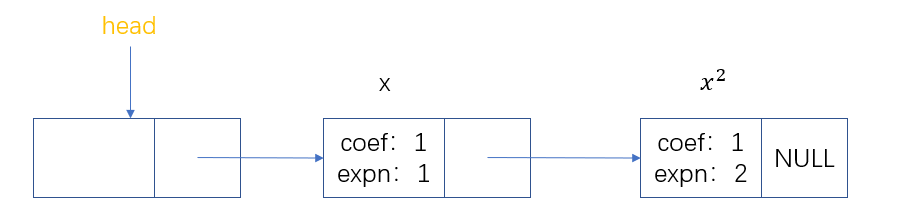一元稀疏多项式链表构建代码：

printf("请输入项数：");
int n;
scanf("%d",&n);//获取项数

for(int i=1;i<=n;i++){
Polynomial *p = (Polynomial *)malloc(sizeof(Polynomial));
printf("请输入第%d项的系数和指数：",i);
scanf("%f %d",&p->coef,&p->expn);
if(p->coef == 0)//系数为0，不存储
free(p);
else{
Polynomial *p1, *p2;//两个指针一前一后便于操作

while(p2 != NULL && p2->expn < p->expn){//找到指数大于p的 / 或者为空
p1 = p2;
p2 = p2->next;
}
if(p2 != NULL && p2->expn == p->expn){//指数相等，合并
p2->coef += p->coef;
if(p2->coef == 0){//若合并后系数为0，则删除该项
p1->next = p2->next;
free(p2);
}
free(p);
}
else{//不存在指数相等，则添加在p1后面
p->next = p2;
p1->next = p;
}
}
}
}

四、多项式加减法

基本思路：所有的多项式的是进行指数排序的，所以我们可以像单调链表的合并一样操作。

1. 当指数相等时，系数相加（减法时则相减）；相加完的系数若不为0，则存进链表C；
2. 若指数不相等，若A的指数小于B的，将A的节点里的系数，指数都存进链表C；
3. 反之，则将B的节点里的系数，指数都存进链表C；
4. 当有一个链表的后几项多出来时，也将其存进链表C。

多项式加减法的代码实现：index控制加减，1为加，-1为减；A+B或A-B；返回结果链表

while(a2 && b2){//循环比较，指数小的放前面
if(a2->expn < b2->expn){//a2的指数小于b2，则将a2放入c的尾
a1->next = a2->next;//将a2从a中分离出来
a2->next = c1->next;
c1->next = a2;//将a2接在c的尾

a2 = a1->next;//a2指向a1的后一个结点
c1 = c1->next;//c1移动到c的尾（由于刚刚添加了一个，所以往后移一个）
}
else if(a2->expn == b2->expn){//a2和b2指数相同，两者合并，在放入c的尾
a2->coef += index * b2->coef;//系数和，设置index可以控制加减法
if(a2->coef != 0){//合并后系数不为0，需要将合并后的a2加入c
a1->next = a2->next;//将a2从a中分离出来
a2->next = c1->next;
c1->next = a2;//将a2接在c的尾

c1 = c1->next;//c1移动到c的尾（由于刚刚添加了一个，所以往后移一个）
a2 = a1->next;//a2指向a1的后一个节点
b1->next = b2->next;//将b2也分离出来
free(b2);//释放b2的空间
b2 = b1->next;//b2指向b1的后一个节点
}
else{//合并后系数为0，则不需要加入到c中
a1->next = a2->next;//将a2去除
free(a2);//释放a2空间
a2 = a1->next;
b1->next = b2->next;//将b2去除
free(b2);//释放b2空间
b2 = b1->next;
}
}
else{//b2的指数小于a2，则将b2放入c的尾
b1->next = b2->next;//将b2从b中分离出来
b2->next = c1->next;
c1->next = b2;//将b2接在c的尾

b2 = b1->next;//b2指向b1的下一个节点
c1 = c1->next;//c1移动到c的尾（由于刚刚添加了一个，所以往后移一个）
}
}

while(a2){
a2->coef *= index;//减，就让系数变号
a1->next = a2->next;//将a2接在c后面
a2->next = c1->next;
c1->next = a2;
c1 = c1->next;
a2 = a1->next;
}
while(b2){
b2->coef *= index;//减，就让系数变号
b1->next = b2->next;//将b2接在c后面
b2->next = c1->next;
c1->next = b2;
c1 = c1->next;
b2 = b1->next;
}

}

五、多项式相乘

基本思路：按位相乘，循环遍历；时间复杂度n2
A = a1xi1+a2xi2
B = b1xi3+b2xi4
A * B = a1*b1xi1+i3 + a1*b2xi1+i4 + a2*b1xi2+i3 + a2*b2xi2+i4

while(a2){
while(b2){
Polynomial *p = (Polynomial *)malloc(sizeof(Polynomial));
p->coef = a2->coef * b2->coef;//系数相差
p->expn = a2->expn + b2->expn;//指数相加

if(p->coef == 0)//系数为0，不存储
free(p);
else{
Polynomial *p1, *p2;//两个指针一前一后便于操作
p1 = c1;
p2 = c1->next;

while(p2 != NULL && p2->expn < p->expn){//找到指数大于p的 / 或者为空
p1 = p2;
p2 = p2->next;
}
if(p2 != NULL && p2->expn == p->expn){//指数相等，合并
p2->coef += p->coef;
if(p2->coef == 0){//若合并后系数为0，则删除该项
p1->next = p2->next;
free(p2);
}
free(p);
}
else{//不存在指数相等，则添加在p1后面
p->next = p2;
p1->next = p;
}
}
b2 = b2->next;//b2向下移一个
}
a2 = a2->next;//a2向下移一个
}

}

展开全文数据结构
• 本篇博客是B站教学视频的学习笔记，...Matlab多项式与数据统计 % 介绍多项式的内容 p=[1,2,3,4]; f1=poly2str(p,'x'); % 生成好看的符号串 % disp(f1) 结果为 x^3 + 2 x^2 + 3 x + 4 f2=poly2sym(p); % 生成可用的符号.

本篇博客是B站教学视频的学习笔记，视频教程地址：https://www.bilibili.com/video/BV1hE411Q7T4，Up主讲的很好，部分内容有删减。部分内容为我自己的理解，由于本人水平有限，可能存在表述不准确的地方，见谅！

Matlab多项式与数据统计

% 介绍多项式的内容
p=[1,2,3,4];
f1=poly2str(p,'x'); % 生成好看的符号串
% disp(f1) 结果为 x^3 + 2 x^2 + 3 x + 4
f2=poly2sym(p); % 生成可用的符号函数
% disp(f2) 结果为 x^3 + 2*x^2 + 3*x + 4
x=2;
y=polyval(p,x); %带入求值，即求 x^3 + 2*x^2 + 3*x + 4 在x=2时的值。
% 知道系数矩阵，求根
r=roots(p);
% 知道根，求系数矩阵
p_=poly(r);
% 数据插值操作
X=[-3,-1,0,1,3];
Y=[9,1,0,1,9];
% 上面两行描述已知点。
y2=interp1(X,Y,2); % 使用插值方法，预测当x=2时，值为多少
y2m=interp1(X,Y,2,'spline'); %使用三次样条方法插值，估计当x=2时的值为多少。

% 数据统计操作
% 定义数据
X = [2, 3, 9, 15, 6, 7, 4];
A = [1, 7, 2; 9, 5, 3; 8, 4 ,6];
B = [1, 7, 3; 9, 5, 3; 8, 4 ,6];

% 求矩阵的最大值
y=max(X);
[y,k]=max(X); % k为最大值的角标，y为最大值的值
max(A,[],1); % 求每一列的最大值，结果为行向量
max(A,[],2); % 求每一行的最大值，结果为列向量,
[y,k]=max(A,[],1); % 不仅返回最大值，还有角标位置

% 均值和中值
y=mean(X);
mean(A,1); % 求Ａ每一列的均值，结果为行向量
mean(A,2); % 求Ａ每一行的均值，结果为列向量
y=median(X);
median(A,1); % 求A每一列的中值，结果为行向量
median(A,2); % 求A每一行的中值，结果为列向量

% 其它运算
y = sum(X); % 求和
y = prod(X); % 求积
y = cumsum(X); % 累加
y = cumprod(X); % 累乘

% 排序操作
% sort(矩阵, dim, 'method') dim为1按列排序，2按行排序；
% ascend升序，descend降序
Y = sort(A, 1, 'ascend');
[Y,I] = sort(A, 1, 'ascend'); % I表示Y中对应元素在原来数组中的角标

Matlab符号函数

% 符号函数创建
% 使用sym函数
p=sin(pi/3); % p = 0.8660
% p:符号常量
% 第二个参数：d:浮点数，f:有理分数，e:有理数和误差，r:有理数
P=sym(p,'r'); % 用数值创建符号常量P；

%使用syms函数
syms x; %声明符号变量
f=7*x^2+2*x+9; %创建符号函数

% 符号运算
%符号运算
% 加减乘除外
% '转置 ； ==相等 ； ~=不等
% sin, cos, tan; asin, acos, atan 三角反三角
% sinh, cosh, tanh; asinh, acosh, atanh 双曲反双曲
% conj复数共轭；real复数实部；imag复数虚部；abs复数模；angle复数幅角
% diag矩阵对角；triu矩阵上三角；tril矩阵下三角；inv逆矩阵；det行列式；rank秩；poly特征多项式；
% |----expm矩阵指数函数；eig矩阵特征值和特征向量；svd奇异值分解；

% 精度控制
digits; % 显示当前计算精度
digits(16); % 将精度设置为16
a16=vpa(sqrt(2)); % 以16位的精度计算sqrt(2);
a8=vpa(sqrt(2),8); % 以8位的精度计算sqrt(2);

% 符号多项式函数运算
g=expand(f); % 展开
h=collect(g); % 整理，默认按照x整理
h1=collect(f,x); % 按照x整理；
% 因式分解展开质因数
fac=factor(h);
factor(12);
% 符号多项式向量形式与计算
syms a b c; % 定义符号变量
n=[a,b,c]; % 构建符号向量
roots(n);  % 求符号多项式ax^2+b^x+c=0的根
n=[1,2,3];
roots(n); % 求符号多项式带入 a=1,b=2,c=3的根
% 反函数
fi=finverse(f,x);

% 符号函数微积分
limit(f,x,4); % 求关于x的函数在x->4时的极限
limit(f,4); % 默认变量等于4时的极限
limit(f); % 等价于 limit(f,0)
limit(f,x,4,'left'); % 求左极限，x->4-

% 基本级数运算
syms x;
s=x+1;
symsum(s,x,[0 2]); % 结果是6，分别将0,1,2 带入x+1并求和
symsum(s,x,0,2); %与上面等价
symsum(s,x,[0;2]) % 与上面等价
% 一维泰勒展开
taylor(f,x,4); % 在x=4处展开为5阶泰勒级数
taylor(f,4); % 在默认变量=4处展开为5阶泰勒级数
taylor(f); % 在默认变量=0处展开为5阶泰勒级数

% 符号微分
n=1;
fn=diff(f,x,n); % f(x) 对x的n阶导
diff(f,1);
diff(f);

% 多元偏导
fxy=diff(f,x,y); % 先求x偏导，再求y偏导
fxyz=diff(f,x,y,z); % 先求x偏导，再求y偏导，最后求z偏导

% 符号积分
% 定积分
int(s,x,1,2);
int(s,1,2);
% 不定积分
int(s,x);
int(s);

% 符号方程求解
% 一元方程
eqn1=a*x==b;
S=solve(eqn1);
% 多元方程组
eqn21 = x-y==a;
eqn22 = 2*x+y==b;
% [Svar1,...SvarN]=solve(eqn1,...eqnM, var1,...varN),MN不一定相等
[Sx, Sy] = solve(eqn21, eqn22, x, y);
% 加上参数ReturnCondition可返回通解及解的条件
[Sxn, Syn] = solve(eqn21, eqn22, x, y, 'ReturnCondition', true);
% 其他参数(参数加上true生效)
% IgnoreProperty，忽略变量定义时一些假设
% IgnoreAnalyticConstraints，忽略分析限制；
% MaxDegree，大于3解显性解；
% PrincipleValue，仅主值
% Real，仅实数解

展开全文• //若是用L来遍历b，我们每次循环后都要将已经处理过的b的元素删掉（避免重复添加） //这样就会出现重加（b的这个元素与L的这个元素指数不等，但是可能会有L的其它元素与b的这个元素相等） //不好判断是否删除b元素...c语言 链表 开发语言 1024程序员节
• 多项式环

千次阅读 2018-11-22 09:53:47
对于整环RRR，在RRR上定义一个含有为定元xxx的多项式 f(x)=anxn+an−1xn−1+...+a1x+a0,n≥0f(x)=a_nx^n+a_{n-1}x^{n-1}+...+a_1x+a_0,n \ge 0f(x)=an​xn+an−1​xn−1+...+a1​x+a0​,n≥0mmm是使得am≠0a_m \neq0...数学 多项式
• 极小多项式

千次阅读 2017-05-07 19:48:00
极小多项式定义：适合矩阵A的最小次数的多项式 最下多项式一定存在且唯一 纯量矩阵的最小多项式 如果A可对角化，则其极小多项式没有重根---如果矩阵A的极小多项式没有重根---则矩阵A可以对角化 ...
• 机器学习算法之多项式回归

千次阅读 2019-05-12 21:05:14
机器学习算法之多项式回归 目录: 1.多项式回归简介 2.编程实验多项式回归 3.过拟合和欠拟合 4.学习曲线 5.验证数据集与交叉验证 6.偏差方差均衡 7.模型正则化 一、多项式回归简介 考虑下面的数据，虽然我们可以...机器学习算法 多项式回归
• 多项式 什么是多项式 满足如下条件的表达式才是多项式: 1 包含变量或者变量与常量 2 涉及的运算只有加运行，减运算，乘法运算与指数运算(指数必须&gt;=0，不可以是负数)，不包含除法运算   线性多项式 多项式中...
• c语言实现多项式的基本运算

千次阅读 2020-09-22 23:32:35
多项式是数学中常用的一种表达式，现在我们给出用c语言编程实现多项式的计算，并且多项式的计算是链表的典型应用，通过编程实现多项式，也为我们巩固一下链表的知识以及它的生活应用。 下面给出代码 #include <...链表 指针 数据结构 c语言
• 两个多项式相加——C语言

千次阅读 多人点赞 2018-07-08 09:26:26
#include &lt;stdio.h&gt; #include &lt;stdlib.h&gt; typedef struct Node { int coef;//系数 int expn;//指数 struct Node *next;... //LNode为创建的多项式链表类型 struct elem { int ...链表 数据结构
• 数据结构之一元多项式

千次阅读 2014-03-20 01:05:43
上个星期完成了老师布置的作业———《数据结构》的顺序表和一元多项式，本打算前两天写关于顺序表的博客文章，但后来想了想，还是写这个链表的吧。当你们按照书上的代码敲上去vc或者其他编译器时候，是不是发现无法...
• 一.一元多项式的根(7.6) 二.复数域上的不可约多项式(7.6) 三.实数域上的不可约多项式(7.7) 四.实数系多项式的根(7.7)不可约多项式 多项式的根
• 二元多项式

千次阅读 2017-08-22 17:16:15
给你多个二元多项式和一个操作符，让你输出操作符操作这些二元多项式之后的结果。 Input 首先输入二元多项式的个数n和操作符号（‘+’，‘*’）； 后面n行输入每一个多项式。 多组输入，当n=0的时候结束输入。 ...
• 多项式

千次阅读 2013-09-17 14:18:32
多项式中每一个 x n 皆称之为多项式的项 次数：多项式 x n 中每一项的n为此项的次数 同次项：若有多个多项式，其中每一项的 x k 项称之为同次项 首项：指多项式的项中次数最大者，若多项式首项为n，则称此多项式为n...数学 多项式 算法 编程
• #数据结构 Record Two# (多项式的相加和相乘)首先还是从一道题入手来引出多项式相乘和相加的算法思想，下面请看题：&lt;&lt;&lt;题目&gt;&gt;&gt;02-线性结构2 一元多项式的乘法与加法运算...
• 三次、五次多项式

千次阅读 2019-12-31 10:11:49
• 要求一高阶多项式的根往 往须借助数值方法,所 幸MATLAB已将这些数值方法写成一函数 roots(p),我们只要输入多项式的各阶系数 (以 p 代表)即可求解到对应的根 >......2. polyvalm函数用来求矩阵多项式的值,其中参数...
• 多项式轨迹–三次轨迹 1.4 Cubic trajectory 图 3 三次多项式轨迹 一旦指定了t0,t1{{t}_{0}},{{t}_{1}}t0​,t1​时刻的位置和速度的值(q0,q1v0,v1)\left({{q}_{0}},{{q}_{1}}{{v}_{0}},{{v}_{1}} \right)(q0​,q1​...三次多项式 多项式轨迹 速度规划 多项式插值
• 一、函数的近似表示—高次多项式 二、误差函数—最小二乘法 三、引出案例函数曲线 四、目标函数 五、优化目标函数 六、优化目标函数—梯度下降法 七、优化目标函数—求解线性方程组 八、python编程实现拟合...最小二乘法
• 一.n元多项式环 1.nnn元多项式的概念 2.nnn元多项式的运算 3.数域KKK上nnn元多项式K[x1,x2...xn]K[x_1,x_2...1.nnn元对称多项式定义 2.数域KKK上nnn元对称多项式组成的集合的结构 3.数域KKK上一元多项式的判别式 ...n元对称多项式
• 文章目录多元多项式**n** 元多项式概念n元多项式n元多项式环nnn 元多项式的字典排列法有关性质齐次多项式nnn 元多项式函数对称多项式一元多项式根与系数的关系nnn 元对称多项式例1例2 \quad一 元多项式的判别式例3...线性规划 自然语言处理 数据挖掘 机器学习 深度学习
•  定义多项式的结构为线性链表的存储结构，每个结点包含三个元素：系数coef，指数expn和指向下一个结点的指针*next。通过指针，我们就可以把多个单项式连接起来，形式一个多项式(单项式也是多项式)。 （2）建立...
• 多项式插值 先有一个函数f(x)f(x)f(x)，如果给定在区间[a,b][a,b][a,b]上的n+1n+1n+1个点a&amp;lt;=x0&amp;lt;x1&amp;lt;⋅⋅⋅&amp;lt;xn&amp;lt;=ba&amp;lt;=x_0&amp;lt;x_1&amp;...
• 数据结构实践——链表：多项式求和

万次阅读 多人点赞 2015-09-12 11:31:31
本文针对数据结构基础系列网络课程(2)：线性表的实践项目。【项目6 - 多项式求和】 　用单链表存储一元多项式，并实现两个多项式的加法。 提示： 1、存储多项式的数据结构 ...直观地，可以定义一个数数据结构 链表 多项式
• 数据结构—— 一元多项式的加法运算

万次阅读 多人点赞 2019-09-24 12:01:56
设Pn(x)和Qn(x)分别为两个一元多项式，请求出两个一元多项式的加法运算的结果，要求元素按照多项式的次数递减的次序排列。 1.问题分析 需求：实现两个一元多项式的加法运算。 实现功能：①通过键盘接收输入的整数...数据结构 一元多项式加法运算 线性表应用
• 线性表实现一元多项式操作

千次阅读 多人点赞 2018-10-09 16:34:19
有了思路，我们很容易定义结构 typedef struct node{ float * coef;//系数数组 int maxSize;//最大容量 int order;//最高阶数 }Polynomial; 先实现求和：我们想求两个式子a+b，结果存在c中。 逻辑...
• 包括：多项式的class定义+多项式加法+多项式乘法 黄金周即将过去，好多任务都没有完成，还好提前意识到，最近两天只好奋笔疾书了！ 本篇依旧是数据结构的内容，但是是单链表的一个应用实例，还是比较实用的，相信...单链表的应用 LNK2005
• 一元多项式的建立及其运算

千次阅读 2016-02-05 14:28:10
在表处理时经常遇到的问题是多项式的表示和运算。所以如何建立一个符合多项式的结构是十分重要的。 （一）一元多项式的表示：  一种方法是用顺序表表示，即顺序表的物理位置存储的是多项式中x的指数，而顺序表中......